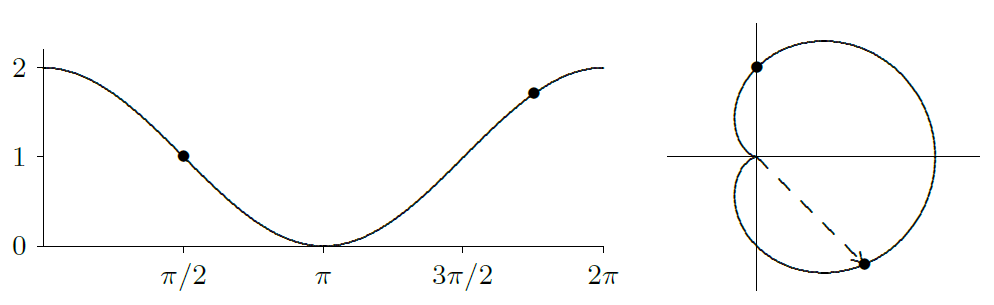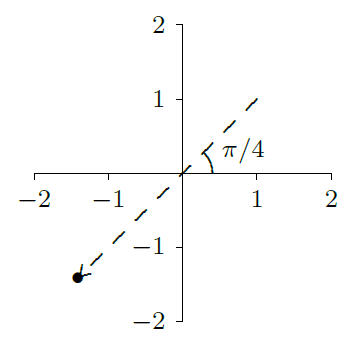$$\newcommand{\id}{\mathrm{id}}$$ $$\newcommand{\Span}{\mathrm{span}}$$ $$\newcommand{\kernel}{\mathrm{null}\,}$$ $$\newcommand{\range}{\mathrm{range}\,}$$ $$\newcommand{\RealPart}{\mathrm{Re}}$$ $$\newcommand{\ImaginaryPart}{\mathrm{Im}}$$ $$\newcommand{\Argument}{\mathrm{Arg}}$$ $$\newcommand{\norm}{\| #1 \|}$$ $$\newcommand{\inner}{\langle #1, #2 \rangle}$$ $$\newcommand{\Span}{\mathrm{span}}$$

# 10.1: Polar Coordinates

•• Contributed by David Guichard
• Professor (Mathematics) at Whitman College

$$\newcommand{\vecs}{\overset { \rightharpoonup} {\mathbf{#1}} }$$

$$\newcommand{\vecd}{\overset{-\!-\!\rightharpoonup}{\vphantom{a}\smash {#1}}}$$

Coordinate systems are tools that let us use algebraic methods to understand geometry. While the rectangular (also called Cartesian) coordinates that we have been using are the most common, some problems are easier to analyze in alternate coordinate systems.

A coordinate system is a scheme that allows us to identify any point in the plane or in three-dimensional space by a set of numbers. In rectangular coordinates these numbers are interpreted, roughly speaking, as the lengths of the sides of a rectangle. In polar coordinates a point in the plane is identified by a pair of numbers $$(r,\theta)$$. The number $$\theta$$ measures the angle between the positive $$x$$-axis and a ray that goes through the point, as shown in figure 10.1.1; the number $$r$$ measures the distance from the origin to the point. Figure 10.1.1 shows the point with rectangular coordinates $$(1,\sqrt3)$$ and polar coordinates $$(2,\pi/3)$$, 2 units from the origin and $$\pi/3$$ radians from the positive $$x$$-axis.Figure 10.1.1. Polar coordinates of the point $$(1,\sqrt3)$$.

Just as we describe curves in the plane using equations involving $$x$$ and $$y$$, so can we describe curves using equations involving $$r$$ and $$\theta$$. Most common are equations of the form $$r=f(\theta)$$.

Example 10.1.1

Graph the curve given by $$r=2$$.

Solution

All points with $$r=2$$ are at distance 2 from the origin, so $$r=2$$ describes the circle of radius 2 with center at the origin. Just as we describe curves in the plane using equations involving $$x$$ and $$y$$, so can we describe curves using equations involving $$r$$ and $$\theta$$. Most common are equations of the form $$r=f(\theta)$$.

Example 10.1.2

Graph the curve given by $$r=1+\cos\theta$$.

Solution

We first consider $$y=1+\cos x$$, as in figure 10.1.2. As $$\theta$$ goes through the values in $$[0,2\pi]$$, the value of $$r$$ tracks the value of $$y$$, forming the "cardioid'' shape of figure 10.1.2. For example, when $$\theta=\pi/2$$, $$r=1+\cos(\pi/2)=1$$, so we graph the point at distance 1 from the origin along the positive $$y$$-axis, which is at an angle of $$\pi/2$$ from the positive $$x$$-axis. When $$\theta=7\pi/4$$, $$r=1+\cos(7\pi/4)=1+\sqrt2/2\approx 1.71$$, and the corresponding point appears in the fourth quadrant. This illustrates one of the potential benefits of using polar coordinates: the equation for this curve in rectangular coordinates would be quite complicated.Figure 10.1.2. A cardioid: $$y=1+\cos x$$ on the left, $$r=1+\cos\theta$$ on the right.

Each point in the plane is associated with exactly one pair of numbers in the rectangular coordinate system; each point is associated with an infinite number of pairs in polar coordinates. In the cardioid example, we considered only the range $$0\le \theta\le2\pi$$, and already there was a duplicate: $$(2,0)$$ and $$(2,2\pi)$$ are the same point. Indeed, every value of $$\theta$$ outside the interval $$[0,2\pi)$$ duplicates a point on the curve $$r=1+\cos\theta$$ when $$0\le\theta < 2\pi$$. We can even make sense of polar coordinates like $$(-2,\pi/4)$$: go to the direction $$\pi/4$$ and then move a distance 2 in the opposite direction; see figure 10.1.3. As usual, a negative angle $$\theta$$ means an angle measured clockwise from the positive $$x$$-axis. The point in figure 10.1.3 also has coordinates $$(2,5\pi/4)$$ and $$(2,-3\pi/4)$$.Figure 10.1.3. The point $$(-2,\pi/4)=(2,5\pi/4)=(2,-3\pi/4)$$ in polar coordinates.

The relationship between rectangular and polar coordinates is quite easy to understand. The point with polar coordinates $$(r,\theta)$$ has rectangular coordinates $$x=r\cos\theta$$ and $$y=r\sin\theta$$; this follows immediately from the definition of the sine and cosine functions. Using figure 10.1.3 as an example, the point shown has rectangular coordinates $$x=(-2)\cos(\pi/4)=-\sqrt2\approx 1.4142$$ and $$y=(-2)\sin(\pi/4)=-\sqrt2$$. This makes it very easy to convert equations from rectangular to polar coordinates.

Example 10.1.3

Find the equation of the line $$y=3x+2$$ in polar coordinates.

Solution

We merely substitute: $$r\sin\theta=3r\cos\theta+2$$, or $$r= {2\over \sin\theta-3\cos\theta}$$.

Example 10.1.4

Find the equation of the circle $$(x-1/2)^2+y^2=1/4$$ in polar coordinates.

Solution

Again substituting: $$(r\cos\theta-1/2)^2+r^2\sin^2\theta=1/4$$. A bit of algebra turns this into $$r=\cos(t)$$. You should try plotting a few $$(r,\theta)$$ values to convince yourself that this makes sense.

Example 10.1.5

Graph the polar equation $$r=\theta$$.

Solution

Here the distance from the origin exactly matches the angle, so a bit of thought makes it clear that when $$\theta\ge0$$ we get the spiral of Archimedes in figure 10.1.4. When $$\theta < 0$$, $$r$$ is also negative, and so the full graph is the right hand picture in the figure.Figure 10.1.4. The spiral of Archimedes and the full graph of $$r=\theta$$.

Converting polar equations to rectangular equations can be somewhat trickier, and graphing polar equations directly is also not always easy.

Example 10.1.6

Graph $$r=2\sin\theta$$.

Solution

Because the sine is periodic, we know that we will get the entire curve for values of $$\theta$$ in $$[0,2\pi)$$. As $$\theta$$ runs from 0 to $$\pi/2$$, $$r$$ increases from 0 to 2. Then as $$\theta$$ continues to $$\pi$$, $$r$$ decreases again to 0. When $$\theta$$ runs from $$\pi$$ to $$2\pi$$, $$r$$ is negative, and it is not hard to see that the first part of the curve is simply traced out again, so in fact we get the whole curve for values of $$\theta$$ in $$[0,\pi)$$. Thus, the curve looks something like figure 10.1.5. Now, this suggests that the curve could possibly be a circle, and if it is, it would have to be the circle $$x^2+(y-1)^2=1$$. Having made this guess, we can easily check it. First we substitute for $$x$$ and $$y$$ to get $$(r\cos\theta)^2+(r\sin\theta-1)^2=1$$; expanding and simplifying does indeed turn this into $$r=2\sin\theta$$.Figure 10.1.5. Graph of $$r=2\sin\theta$$.

## Contributors

• Integrated by Justin Marshall.